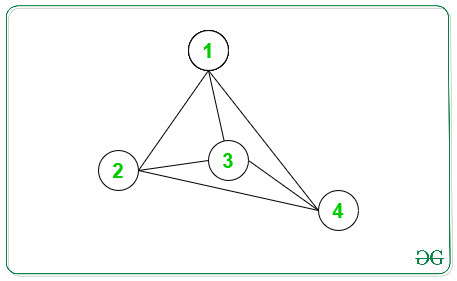# Difference Between sum of degrees of odd and even degree nodes in an Undirected Graph

Given an undirected graph with N vertices and M edges, the task is to find the absolute difference Between sum of degrees of odd degree nodes and even degree nodes in an undirected Graph.

Examples:

Input: N = 4, edges[][] = { { 1, 2 }, { 1, 3 }, { 1, 4 }, { 2, 3 }, { 2, 4 }, { 3, 4 } }
Output: 12
Explanation:
Below is the graph for the above information:Node -> Degree
1 -> 3
2 -> 3
3 -> 3
4 -> 3

Sum of odd degree node = 3 + 3 + 3 + 3 = 12
Sum of even degree node = 0
Difference = 12

Input: N = 5, edges[][] = { { 1, 2 }, { 1, 3 }, { 2, 4 }, { 2, 5 } }
Output: 4

## Recommended: Please try your approach on {IDE} first, before moving on to the solution.

Approach:

1. For each vertex, the degree can be calculated by the length of Adjacency List of the given graph at corresponding vertex.
2. Count the sum of degrees of odd degree nodes and even degree node and print the difference

Below is the implementation of the above approach:

## C++

 `// C++ implementation to print the ` `// Difference Between sum of degrees ` `// of odd degree nodes and even  ` `// degree nodes. ` `#include ` `using` `namespace` `std; ` ` `  `// Function to print the difference ` `// Between sum of degrees of odd ` `// degree nodes and even degree nodes. ` `int` `OddEvenDegree(``int` `N, ``int` `M, ` `                    ``int` `edges[]) ` `{ ` `    ``// To store Adjacency List of ` `    ``// a Graph ` `    ``vector<``int``> Adj[N + 1]; ` `     `  `    ``int` `EvenSum = 0; ` `    ``int` `OddSum = 0; ` ` `  `    ``// Make Adjacency List ` `    ``for` `(``int` `i = 0 ; i < M ; i++) { ` `        ``int` `x = edges[i]; ` `        ``int` `y = edges[i]; ` ` `  `        ``Adj[x].push_back(y); ` `        ``Adj[y].push_back(x); ` `    ``} ` ` `  `    ``// Traverse each vertex ` `    ``for` `(``int` `i = 1; i <= N; i++) { ` ` `  `        ``// Find size of Adjacency List ` `        ``int` `x = Adj[i].size(); ` ` `  `        ``// If length of Adj[i] is ` `        ``// an odd number, add ` `        ``// length in OddSum ` `        ``if` `(x % 2 != 0) ` `        ``{ ` `            ``OddSum += x; ` `        ``} ` `        ``else` `        ``{ ` `            ``// If length of Adj[i] is ` `            ``// an even number, add  ` `            ``// length in EvenSum ` `            ``EvenSum += x; ` `        ``} ` `             `  `    ``} ` `     `  `    ``return` `abs``(OddSum - EvenSum); ` `} ` ` `  `// Driver code ` `int` `main() ` `{ ` `    ``// Vertices and Edges ` `    ``int` `N = 4, M = 6; ` ` `  `    ``// Edges ` `    ``int` `edges[M] = { { 1, 2 }, { 1, 3 }, { 1, 4 }, ` `                       ``{ 2, 3 }, { 2, 4 }, { 3, 4 } }; ` ` `  `    ``// Function Call ` `    ``cout<< OddEvenDegree(N, M, edges); ` ` `  `    ``return` `0; ` `} `

## Java

 `// Java implementation to print the ` `// difference between sum of degrees ` `// of odd degree nodes and even  ` `// degree nodes. ` `import` `java.util.*; ` ` `  `class` `GFG{ ` ` `  `// Function to print the difference ` `// between sum of degrees of odd ` `// degree nodes and even degree nodes. ` `static` `int` `OddEvenDegree(``int` `N, ``int` `M, ` `                         ``int` `edges[][]) ` `{ ` `     `  `    ``// To store adjacency list  ` `    ``// of a graph ` `    ``@SuppressWarnings``(``"unchecked"``) ` `    ``Vector []Adj = ``new` `Vector[N + ``1``]; ` `     `  `    ``for``(``int` `i = ``0``; i < N + ``1``; i++) ` `    ``{ ` `       ``Adj[i] = ``new` `Vector(); ` `    ``} ` `     `  `    ``int` `EvenSum = ``0``; ` `    ``int` `OddSum = ``0``; ` ` `  `    ``// Make adjacency list ` `    ``for``(``int` `i = ``0``; i < M; i++) ` `    ``{ ` `       ``int` `x = edges[i][``0``]; ` `       ``int` `y = edges[i][``1``]; ` `        `  `       ``Adj[x].add(y); ` `       ``Adj[y].add(x); ` `    ``} ` ` `  `    ``// Traverse each vertex ` `    ``for``(``int` `i = ``1``; i <= N; i++) ` `    ``{ ` `         `  `       ``// Find size of adjacency list ` `       ``int` `x = Adj[i].size(); ` `        `  `       ``// If length of Adj[i] is ` `       ``// an odd number, add ` `       ``// length in OddSum ` `       ``if` `(x % ``2` `!= ``0``) ` `       ``{ ` `           ``OddSum += x; ` `       ``} ` `       ``else` `       ``{ ` `            `  `           ``// If length of Adj[i] is ` `           ``// an even number, add  ` `           ``// length in EvenSum ` `           ``EvenSum += x; ` `       ``} ` `    ``} ` `    ``return` `Math.abs(OddSum - EvenSum); ` `} ` ` `  `// Driver code ` `public` `static` `void` `main(String[] args) ` `{ ` `     `  `    ``// Vertices and edges ` `    ``int` `N = ``4``, M = ``6``; ` ` `  `    ``// Edges ` `    ``int` `edges[][] = { { ``1``, ``2` `}, { ``1``, ``3` `}, { ``1``, ``4` `}, ` `                      ``{ ``2``, ``3` `}, { ``2``, ``4` `}, { ``3``, ``4` `} }; ` ` `  `    ``// Function call ` `    ``System.out.print(OddEvenDegree(N, M, edges)); ` `} ` `} ` ` `  `// This code is contributed by PrinciRaj1992 `

Output:

```12
```My Personal Notes arrow_drop_upCheck out this Author's contributed articles.

If you like GeeksforGeeks and would like to contribute, you can also write an article using contribute.geeksforgeeks.org or mail your article to contribute@geeksforgeeks.org. See your article appearing on the GeeksforGeeks main page and help other Geeks.

Please Improve this article if you find anything incorrect by clicking on the "Improve Article" button below.

Improved By : princiraj1992